#Function Repository Resource:

# ProportionsBy

Get the proportion of times that each distinct element appears in a list when evaluated with a given function

Contributed by: Richard Hennigan (Wolfram Research)
 ResourceFunction["ProportionsBy"][{e1,e2,…},f] gives an association whose keys are the distinct values of the f[ei] and whose values give the proportion of times these f[ei] values appear. ResourceFunction["ProportionsBy"][f] represents an operator form of ResourceFunction["ProportionsBy"] that can be applied to an expression.

## Details and Options

ResourceFunction["ProportionsBy"][{e1,e2,},f] gives an association whose keys f[ei] are in the order in which the corresponding ei first appear.
ResourceFunction["ProportionsBy"][f][expr] is equivalent to ResourceFunction["ProportionsBy"][expr,f].
ResourceFunction["ProportionsBy"] also works with heads other than List.
When given an Association, ResourceFunction["ProportionsBy"] uses the Values of the association as input.

## Examples

### Basic Examples

Determine the proportion of a list of integers that is EvenQ:

 In:=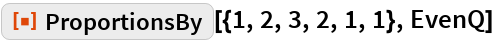Out=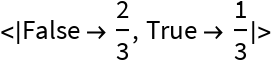Get the proportion of a list that is PrimeQ:

 In:=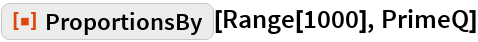Out=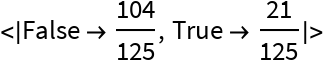Use with an Association:

 In:=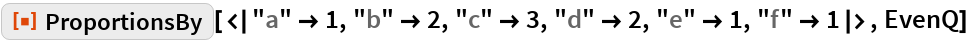Out=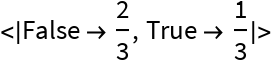Use the operator form of ProportionsBy:

 In:=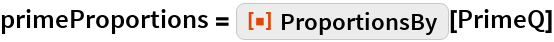Out=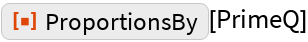In:=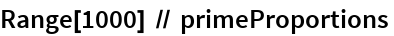Out=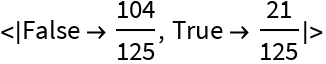### Scope

See how common each character is in a body of text while ignoring case:

 In:=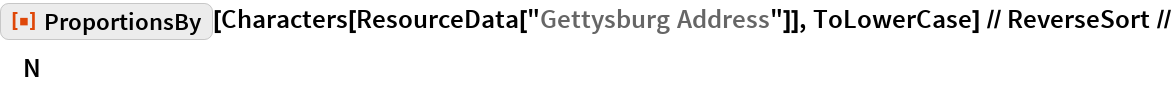Out=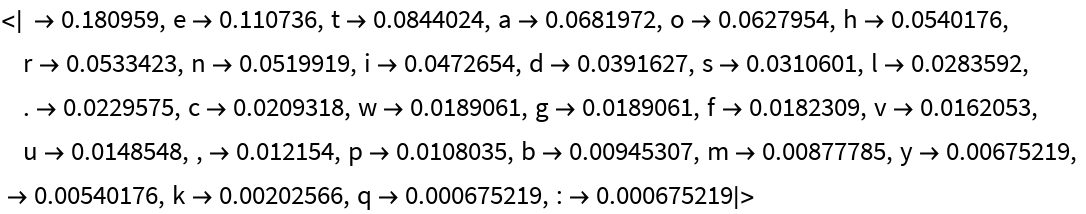Check for common words:

 In:=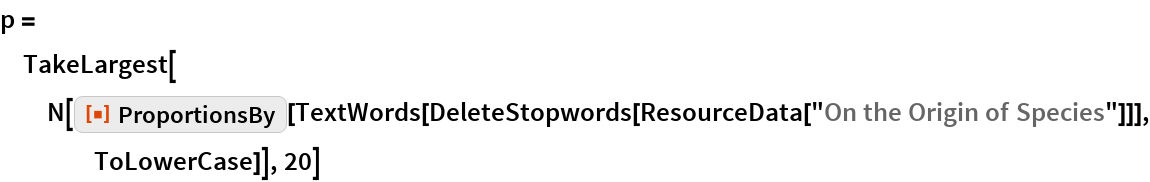Out=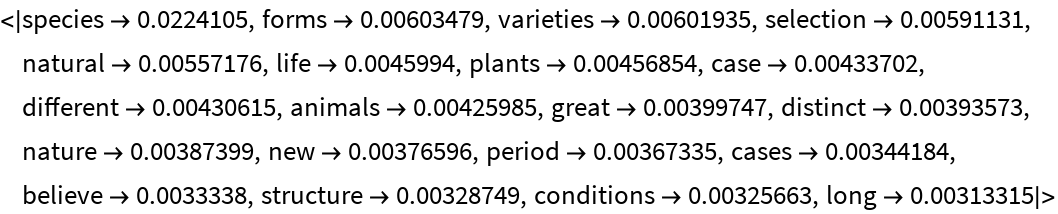In:=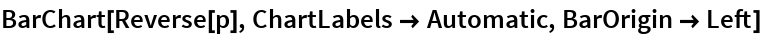Out=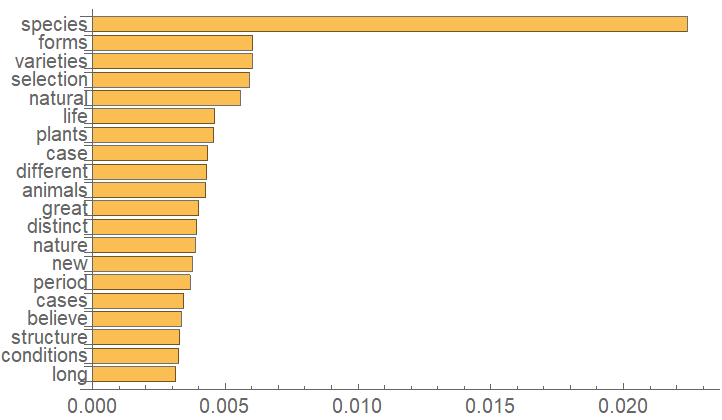Visualize in a WordCloud:

 In:=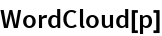Out=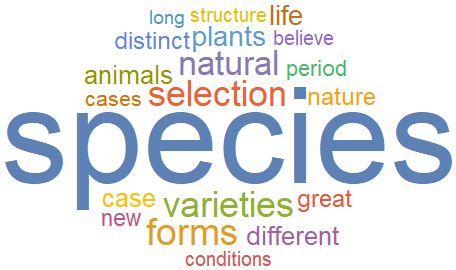### Properties and Relations

The result of ProportionsBy is identical to that of Proportions applied to the results of Map:

 In:=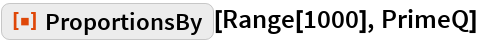Out=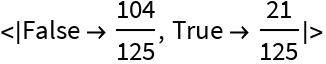In:=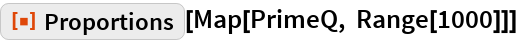Out=## Requirements

Wolfram Language 11.3 (March 2018) or above